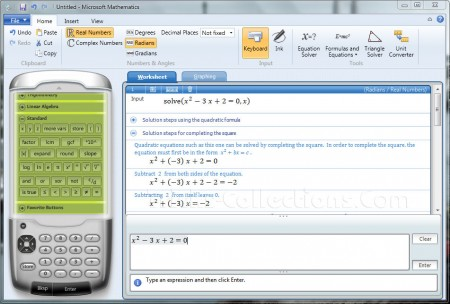You are here:

# Download Microsoft Mathematics – Free Software for Education

Microsoft Mathematics is easy to use software to solve math equation from basic math, algebra, trigonometry, calculus (differential and integral) and more. This software also provides graphing capabilities in Cartesian, parametric and polar and more. This is a great tool for student and teacher. Since Microsoft mathematics version 4.0 this software released as freeware.

The best from Microsoft mathematics, this provides step-by-step instructions the math equation problem solving and explains fundamental concepts. Microsoft Mathematics is standalone software but also can be integrated with Microsoft word using add-ins.

Microsoft Mathematics 4.0 (the latest version) work under windows operating systems: Windows 7, Windows Vista SP2, Windows XP SP3, windows Server 2008.Microsoft Mathematics Features

• Step-by-Step Match Equation Solver to help students learn how to solve math problems
• Graphing calculator to visualize data in two-dimensional and three-dimensional color graphs
• Formulas and Equations Library provides more than 100 commonly used equations and formulae
• Triangle Solver to solve trigonometry-based match equations
• Unit Conversion tool. Easy to use tool to convert units of measure (length, area, volume, weight, temperature, pressure, energy, power, velocity, and time)

The Microsoft Mathematics installer can be downloaded here: download Microsoft mathematics. This software released in January 2011. Useful resource related to Microsoft Mathematics software: Microsoft mathematics teacher guide.

2 Responses

### 2 Responses to “Download Microsoft Mathematics – Free Software for Education”

1. mas awee says:

thanks for information, I’ve tried this software and it works

2. zain says:

thanks for information

Apr
7